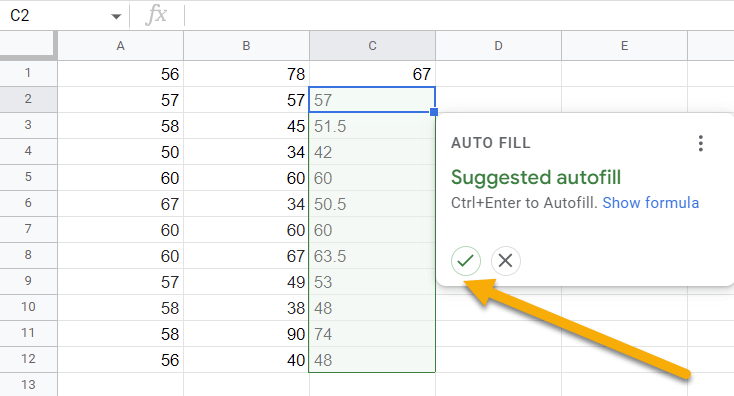# How to☝️ Use the Google Sheets AVERAGE Function

To use the Google Sheets AVERAGE function, click on a blank cell, type the formula =AVERAGE(A1:A12) in the Formula bar, and hit Enter.

This guide covers everything you need to know about the Google Sheets AVERAGE Function: its definition, syntax, use cases, examples, and usage notes.

## How to Quickly Find the Average Value in Google Sheets

The AVERAGE function in Google Sheets is used to calculate the average of a range of cells. Let’s take a look at how to use it!

Difficulty: Beginner

Time Estimate: 5 seconds

1. Select the blank cell where you want the result.
2. Move to the Formula bar and enter the formula =AVERAGE(A1:A12). A1:A12 is our cell range.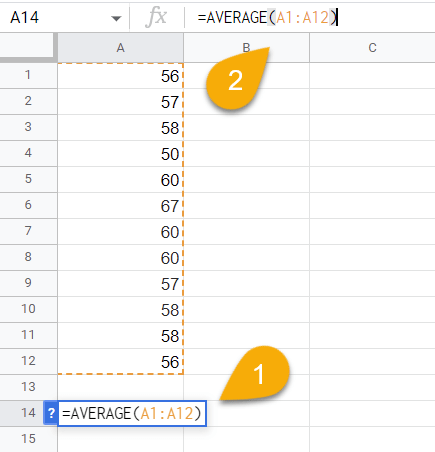1. Press the Enter key on your keyboard.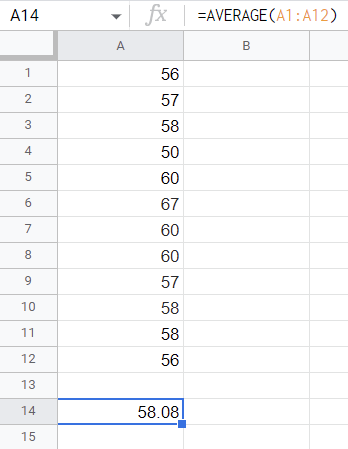Voila! Here is the result!

## Drilling Down into the AVERAGE Function

### Description

When using Google Sheets, you can calculate the average of a range of cells with the AVERAGE function. This function can be used to find the mean of a dataset.

The following example illustrates this: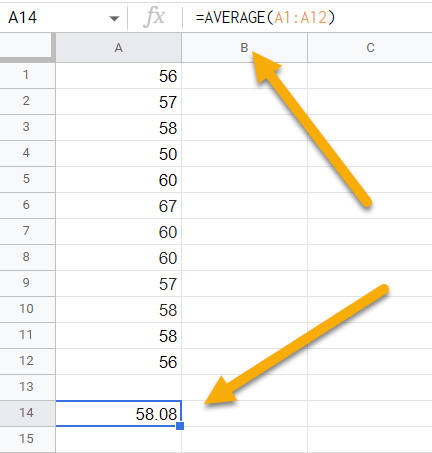### Purpose

The AVERAGE function in Google Sheets can be useful for finding out the average of the numbers in a given range of cells. For example, you can find such things as the average grade in a class or the average cost of an item.

### Syntax

=AVERAGE(value1, [value2, …])

### Arguments

• Value1 and value 2 and so on are the values that are present in the calculation of the average.

### Return Value

Using the AVERAGE function, you will get the average sum of all values.

### Usage Notes

1. The AVERAGE function ignores empty cells and cells with non-numeric values.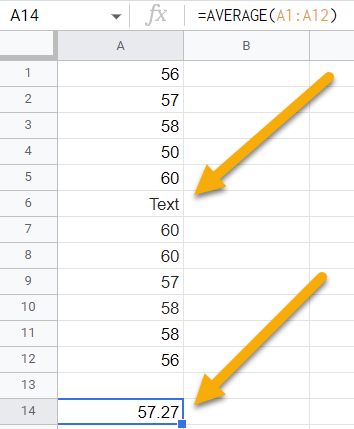2. There is no limit to the number of values you can use with this function.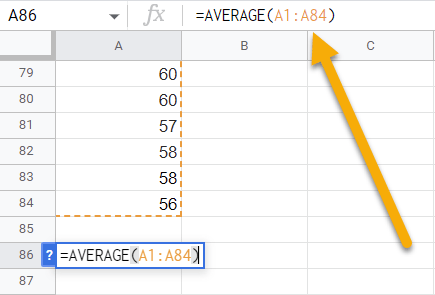### Examples

Here are some examples that we have used while working with this function. In your case, select the values you need for your dataset.

1. If you need to calculate the average with a cell range, use the following formula:

=AVERAGE(A1:A12) // Output: 58.081. You can calculate the average value without using a range of cells by following the formula below:

=AVERAGE(45, 35, 67, 28, 16) // Output: 38. 2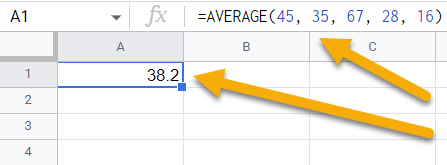## AVERAGE in Google Sheets FAQs

The FAQ below can provide you with more information on this topic if you want to know more.

### How do you average across sheets in Google Sheets?

To average across sheets in Google Sheets, click on the cell where you need the result. In the Formula bar, enter the formula =AVERAGE(A1:A12,Sheet2!A1:A12)—using your own cell range—and press the Enter key on your keyboard.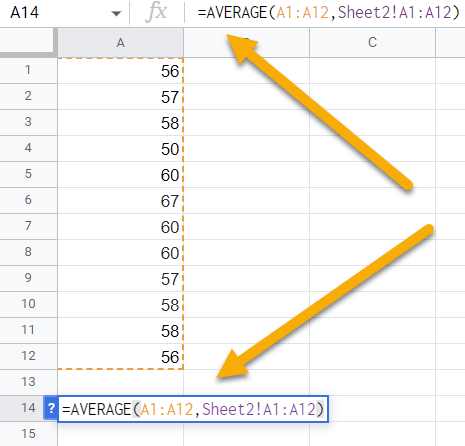### Why is the AVERAGE function not working?

There are a few possible reasons why your AVERAGE function is not working:

• You may have accidentally entered the wrong cell range into the AVERAGE function.
• If your range contains one or more empty cells, this could cause the AVERAGE function to not work.
• One or more of the cells in the range you’re including in the AVERAGE function may contain text instead of a number. The function may not function properly if your range contains text cells.

### How do you calculate the weighted average in Google Sheets?

To calculate the weighted average in Google Sheets, select a blank cell, go to the Formula bar, and enter the formula =AVERAGE.WEIGHTED(A2:A7,B2:B7). A2:A7 and B2:B7 are cell ranges. Finally, hit Enter. Here you can see the result!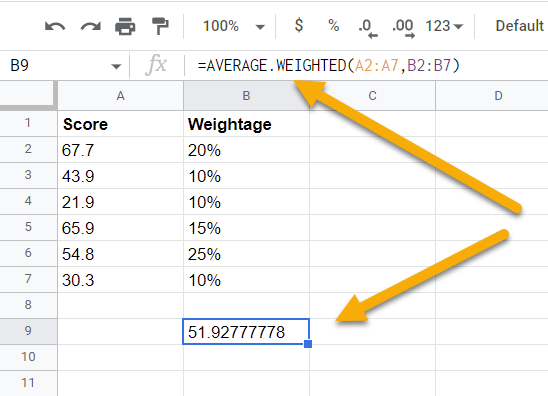### How do I calculate the average of multiple rows in Google Sheets?

To calculate the average of multiple rows in Google Sheets, click on a blank cell, move to the Formula bar, and type the formula =AVERAGE(A1:B1). Here A1:B1 is the cell range we need to calculate, incorporating column A and column B. Then press the Enter key on your keyboard.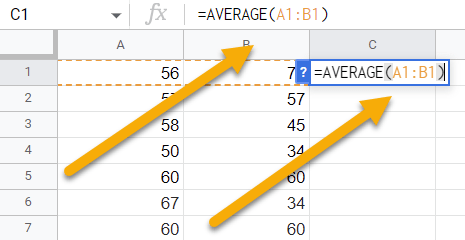Next, click on the check mark to automatically fill all values.